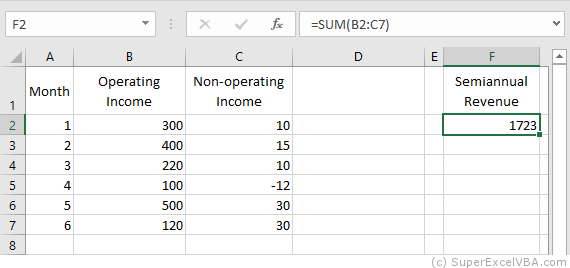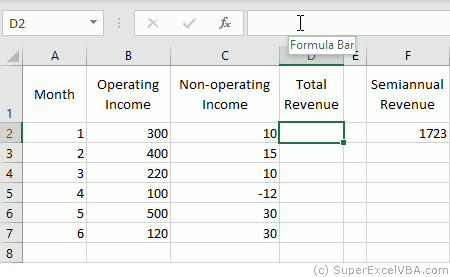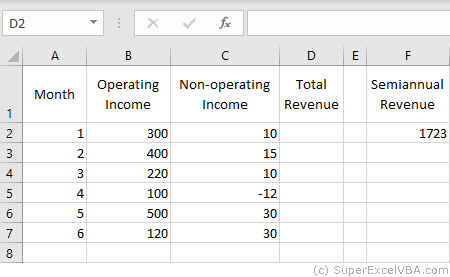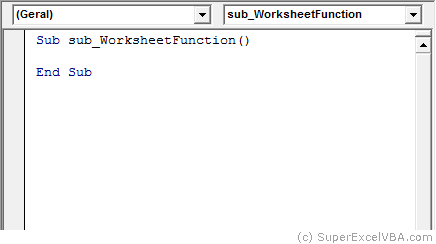# VBA Formulas

It is possible to use Excel's ready-to-use formulas through VBA programming. These are properties that can be used with Range or Cells.

## VBA Formula

Formula adds predefined Excel formulas to the worksheet. These formulas should be written in English even if you have a language pack installed.

    Range("F2").Formula = "=SUM(B2:C7)"
Range("F3").Formula = "=SUM($B$2:$C$7)"Do not worry if the language of your Excel is not English because, as in the example, it will do the translation to the spreadsheet automatically.

### Multiple formulas

You can insert multiple formulas at the same time using the Formula property. To do this, simply define a Range object that is larger than a single cell, and the predefined formula will be "dragged" across the range.

"Dragging" manually:"Dragging" by VBA:

    Range("D2:D7").Formula = "=SUM(B2:C2)"Another way to perform the same action would be using FillDown method.

    Range("D2").Formula = "=SUM(B2:C2)"
Range("D2:D7").FillDown


## VBA FormulaLocal

FormulaLocal adds predefined Excel formulas to the worksheet. These formulas, however, should be written in the local language of Excel (in the case of Brazil, in Portuguese).

    Range("F2").FormulaLocal = "=SOMA(B2:C7)"


Just as the Formula property, FormulaLocal can be used to make multiple formulas.

## VBA FormulaR1C1

FormulaR1C1, as well as Formula and FormulaLocal, also adds pre-defined Excel formulas to the spreadsheet; however, the use of relative and absolute notations have different rules. The formula used must be written in English.

FormulaR1C1 is the way to use Excel's ready-to-use formulas in VBA by easily integrating them into loops and counting variables.

In the notations:

• R refers to rows, in the case of vertical displacement
• C refers to columns, in the case of horizontal displacement
• N symbolizes an integer that indicates how much must be shifted in number of rows and/or columns
• Relative notation: Use as reference the Range that called it

The format of the relative formula is: R[N]C[N]:R[N]C[N].

    Range("F2").FormulaR1C1 = "=SUM(RC[-4]:RC[-3])" 'Equals the bottom row
Range("F2").FormulaR1C1 = "=SUM(RC[-4]:RC[-3])"


When N is omitted, the value 0 is assumed.

In the example, RC[-4]:RC[-3] results in "B2: C7". These cells are obtained by: receding 4 columns to the left RC[-4] from Range("F2") to obtain "B2"; and 5 lines down and 3 columns to the left RC[-3] from Range("F2") to obtain "C7".

• Absolute notation: Use the start of the spreadsheet as a reference

The format of the relative formula is: RNCN:RNCN.

    Range("F2").FormulaR1C1 = "=SUM(R2C2:R7C3)" 'Results in "$B$2:$C$7"


N negative can only be used in relative notation.

The two notations (relative and absolute) can be merged.

    Range("F2").FormulaR1C1 = "=SUM(RC[-4]:R7C3)" 'Results in "B2:$C$7"


## VBA WorksheetFunction

Excel formulas can also be accessed by object WorksheetFunction methods.

    Range("F2") = WorksheetFunction.Sum(Range("B2:C7"))


Excel formulas can also be accessed similarly to functions created in VBA.

The formulas present in the WorksheetFunction object are all in English.

One of the great advantages of accessing Excel formulas this way is to be able to use them more easily in the VBA environment.

    MsgBox (WorksheetFunction.Sum(3, 4, 5))
Expense=4
MsgBox (WorksheetFunction.Sum(3, 4, 5,-Expense))


To list the available Excel formulas in this format, simply type WorksheetFunction. that automatically an option menu with all formulas will appear: